FEATool Multiphysics  v1.11Finite Element Analysis Toolbox
Grid

After a model geometry has been defined, a computational grid or mesh must be generated to allow for the finite element discretization. This section describes how to create or import a compatible grid.

# Grid Mode

Grid mode can be selected by pressing the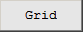mode button or corresponding menu option. In grid mode the toolbar buttons allow for grid generation, refinement, and accessing the advanced grid generation settings.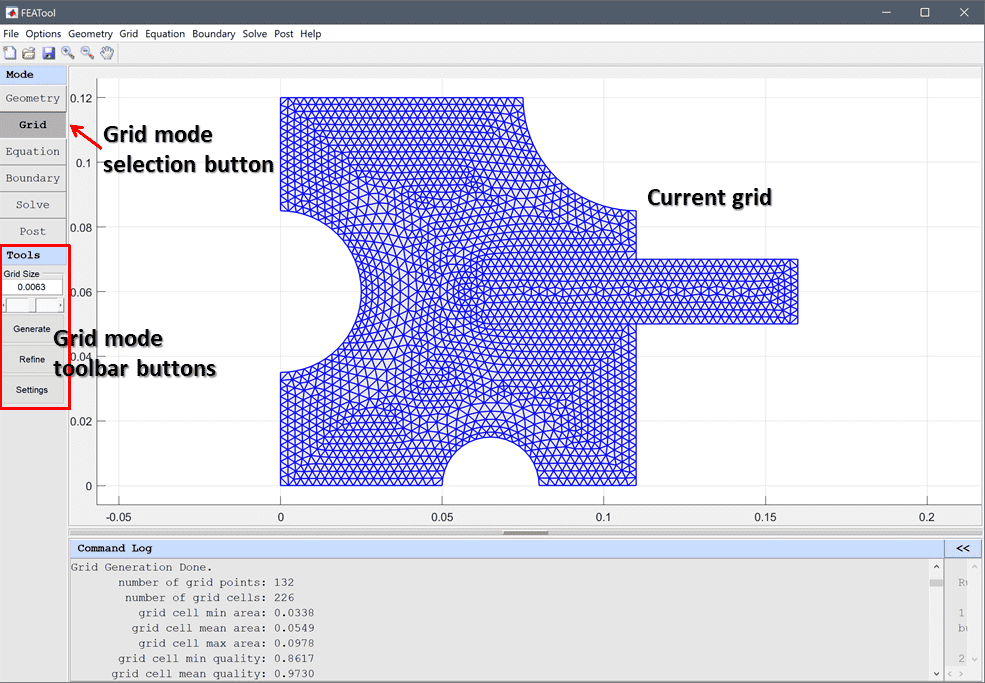•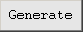calls the selected grid generation function to generate and unstructured. The desired overall mean Grid Size is specified in the corresponding edit field which can be set manually or with the slider control.
• The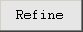button is used to uniformly refine a grid. This will split triangular and quadrilateral (2D) grid cells into four subcells, and tetrahedral and hexahedral (3D) ones into eight subcells each. Grid points on boundaries will also be projected and aligned with any geometries in 2D.
• Advanced grid settings can be controlled by pressing the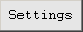button which opens the Grid Generation Settings dialog box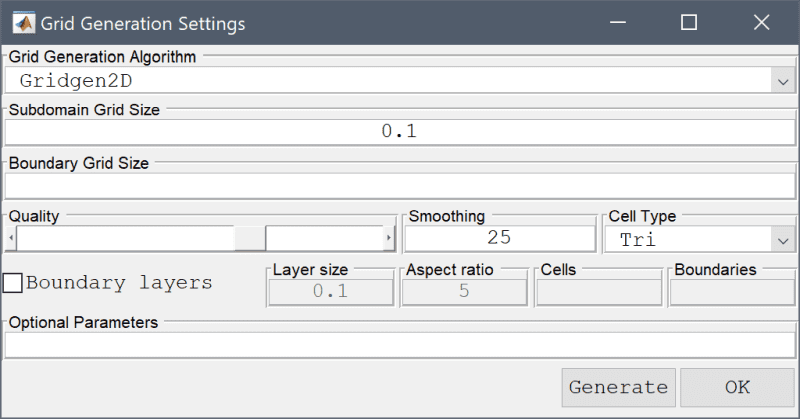The grid settings allows selecting the Grid Generation Algorithm (built-in algorithm, Gmsh, Gridgen2D, or Triangle). The Subdomain Grid Size and Boundary Grid Size edit fields can be used to prescribe maximum grid sizes for subdomains and boundaries. The Quality slider allows setting the target mesh quality. The number of grid Smoothing steps can be specified in the corresponding edit field. Boundary Layers enables generation of structured cells along internal boundaries (holes) for Gridgen2D. Layer Size prescribes the length/size of the boundary layer compared to the overall geometry size, and Aspect Ratio defines the size ratio of boundary layer to normal grid cells. Marking the Internal Boundaries checkbox enables boundary conditions on boundaries between subdomains. And lastly, the Optional Parameters fields allows for an additional string of property/value pairs to be supplied to the grid generation call.

The Grid menu options allows for accessing and specifying the Grid Generation Command... which is a MATLAB function call to support external grid generation tools instead of the default one. Convert Grid Cells is used to convert between triangular and quadrilateral cells in 2D, and between tetrahedral and hexahedral cells in 3D. Grid Statistics... shows statistics, such as grid cell quality,for the current grid.

The Grid menu also supports the following import and export of grids through external ASCII format files

• Import Grid > FEniCS/Dolfin Format... imports a grid in FEniCS/Dolfin format (.xml file) (calls impexp_dolfin).
• Import Grid > GiD Format... imports a grid in GiD format (.msh file) (calls impexp_gid).
• Import Grid > Gmsh Format... imports a grid in Gmsh format (.msh file) (calls impexp_gmsh).
• Import Grid > GMV Format... imports a grid in General Mesh Viewer (GMV) format (.gmv file) (calls impexp_gmv).
• Import Grid > Triangle Format... imports a 2D Triangle grid (.node/.ele files) (calls impexp_triangle).
• Import Grid > VTK Format... imports a ParaView compatible VTK format grid (.vtk file) (calls impexp_vtk).

• Export Grid > FEniCS/Dolfin Format... exports a grid in FEniCS/Dolfin format (.xml file) (calls impexp_dolfin).
• Export Grid > GiD Format... exports a grid in GiD format (.msh file) (calls impexp_gid).
• Export Grid > Gmsh Format... exports a grid in Gmsh format (.msh file) (calls impexp_gmsh).
• Export Grid > GMV Format... exports a grid in General Mesh Viewer (GMV) format (.gmv file) (calls impexp_gmv).
• Export Grid > OpenFOAM Format... exports a grid in OpenFOAM format (calls grid2foam).
• Export Grid > STL Format... exports a STL grid file (.stl file) (calls impexp_stl).
• Export Grid > Triangle Format... exports a 2D Triangle polygonal geometry description (.poly file) (calls impexp_triangle).
• Export Grid > VTK Format... exports a ParaView compatible VTK format grid (.vtk file) (calls impexp_vtk).

# External Grid Generators

FEATool Multiphysics comes with built-in support for the external mesh generators Gmsh (recommended version 3.0.6), Gridgen2D (included with the FEATool distribution), and Triangle (version 1.6). External grid generators typically allows for faster and more robust grid generation, and also supports specifying different grid sizes on subdomains and boundaries. The Grid Generation Algorithm can be selected with the corresponding option in the Grid Generation Settings dialog box.

## Gmsh and Triangle Installation

If Gmsh or Triangle is selected as grid generation algorithm and FEATool cannot find the corresponding binaries, they will automatically be downloaded, and installed when an internet connection is available. Alternatively, the mesh generator binaries can downloaded from the external grid generators repository and/or compiled manually and added to any of the directories available on the MATLAB paths so they can be located by the FEATool program (external binaries are typically placed in the bin folder of the FEATool installation directory). (The grid generator binaries are expected to have the file extensions .exe, _lnx, _mac for Windows, Linux, and Mac OSX platforms respectively.)

## Usage Notes

• Gmsh, Gridgen2D, and Triangle can be used in the FEATool GUI as well as on the MATLAB command line by using the gridgen function with the grid generator arguments gmsh, gridgen2d and triangle, respectively.
• External grid generators works by exporting the current FEATool geometry in its own native format, make a system call to the grid generator binary, and then re-import the generated grid.
• For geometries with multiple and overlapping geometry objects the actual subdomain numbering will generally not correspond to the geometry object numbering (two intersecting geometry objects will for example create three or more subdomains and several internal boundaries). In this case the actual subdomain and boundary numbering for vector valued hmax and hmaxb arrays can easiest be visualized and determined by first creating a coarse grid and switching to Equation/Subdomain and Boundary modes, respectively.
• Gmsh propagates the hmax and hmaxb values down to the specific nodes in the mesh which means that it is currently not possible to exactly define mesh sizes for subdomains and boundaries.

# Grid Reference Material

This section describes the format of the grid data structure that FEATool employs as well as advanced command line (CLI) usage such as manually creating and importing grids.

## Grid Format

The grid format used by FEATool is specified in the grid struct with the following fields

FieldDescriptionSize
pGrid point coordinates(n_sdim, n_points)
cGrid cell connectivity(n_edges_per_cell, n_cells)
aGrid cell adjacency(n_edges_per_cell, n_cells)
bBoundary information(3+n_sdim, n_boundary_points)
sGrid cell subdomain list(1, n_cells)

The coordinates of the grid points are specified by an array p, with the row number indicating the i-th coordinate direction, and column number the corresponding grid point number.

Cell connectivities are specified in the c array, where each column point to the grid points (in p) making up each cell. The row index gives the local vertex number and the column index the cell number. Moreover, the grid points are numbered counterclockwise in each cell.

Adjacency, meaning pointers to neighboring cells, are specified in the a array. Similar to c the row index gives the local edge or face number (starting with the corresponding grid point in c) and the column index points to the cell number. If the edge is on an external boundary the corresponding entry in a is 0.

Boundary information is specified in the b array. The cell, edge/face, and boundary numbers are prescribed in the first to third rows. The last n_sdim rows consists of the outward pointing unit normals corresponding to each boundary cell edge (or face in 3D).

A list of subdomain numbers for each cell is given in the s vector.

## Grid Cell Definitions

The various grid cells available in FEATool are defined in this sub section.

### 1D Line

In one dimension only the simple straight line segment grid cell is available. Grid Example 1 shows how this can be defined and used.

### 2D Triangle (Simplex)

The 2D triangular grid cell is defined with vertices in counter clockwise order. Local edges ei are defined by the local vertices vi as

[ e1 ;    [ v1 v2 ;
e2 ; =    v2 v3 ;
e3 ]      v3 v1 ];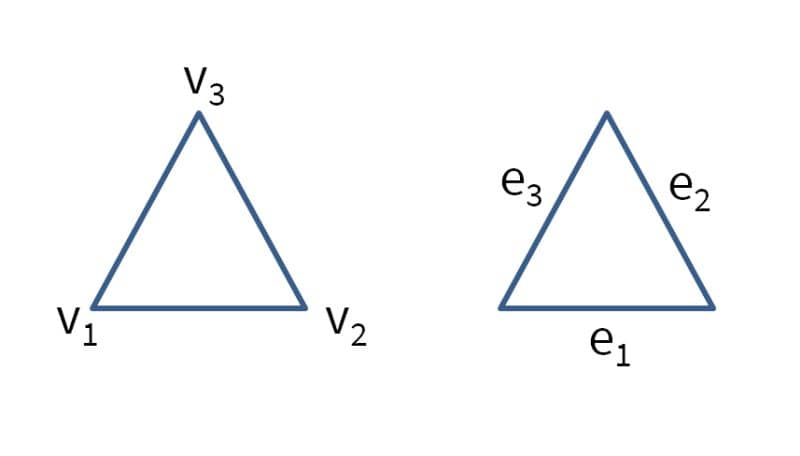The quadrilateral grid cell is similarly defined with vertices in counter clockwise order. Local edges ei are defined by the local vertices vi as

[ e1 ;    [ v1 v2 ;
e2 ;      v2 v3 ;
e3 ] =    v3 v4 ;
e4 ]      v4 v1 ];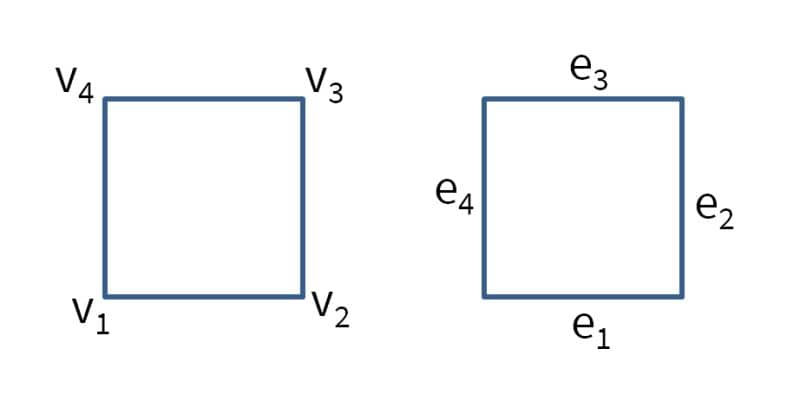### 3D Tetrahedron (Simplex)

The 3D tetrahedral grid cell is defined with vertices in counter clockwise order. Local edges ei are defined by the local vertices vi as

[ e1 ;    [ v1 v2 ;
e2 ;      v2 v3 ;
e3 ;      v3 v1 ;
e4 ; =    v1 v4 ;
e5 ;      v2 v4 ;
e6 ]      v3 v4 ];


The local faces fi are defined by the local vertices vi as

[ f1 ;    [ v1 v2 v3 ;
f2 ;      v1 v2 v4 ;
f3 ; =    v2 v3 v4 ;
f4 ]      v3 v1 v4 ];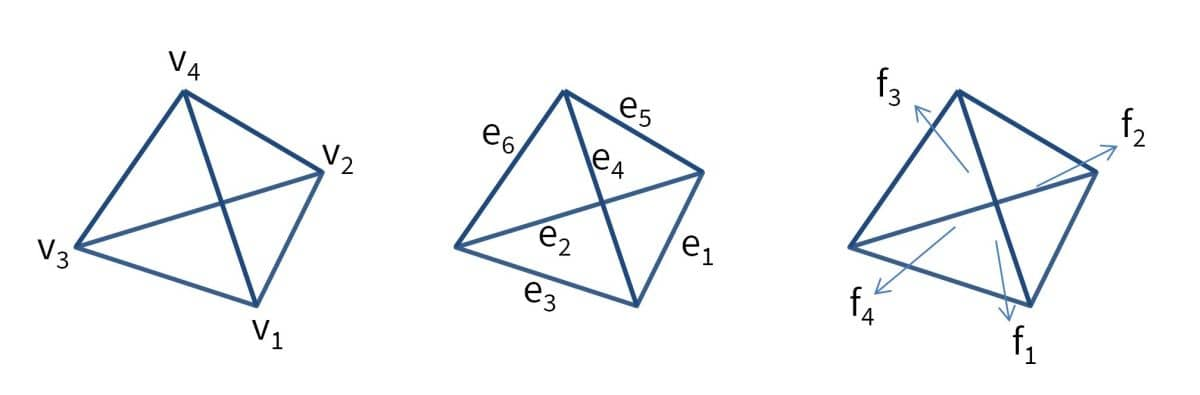### 3D Hexahedron

The hexahedral brick grid cell is defined with vertices in counter clockwise order. Local edges ei are defined by the local vertices vi as

[ e1  ;    [ v1 v2 ;
e2  ;      v2 v3 ;
e3  ;      v3 v4 ;
e4  ;      v4 v1 ;
e5  ;      v1 v5 ;
e6  ;      v2 v6 ;
e7  ; =    v3 v7 ;
e8  ;      v4 v8 ;
e9  ;      v5 v6 ;
e10 ;      v6 v7 ;
e11 ;      v7 v8 ;
e12 ]      v8 v1 ];


The local faces fi are defined by the local vertices vi as

[ f1 ;    [ v1 v2 v3 v4 ;
f2 ;      v1 v2 v6 v5 ;
f3 ;      v2 v3 v7 v6 ;
f4 ; =    v3 v4 v8 v7 ;
f5 ;      v4 v1 v5 v8 ;
f6 ]      v5 v6 v7 v8 ];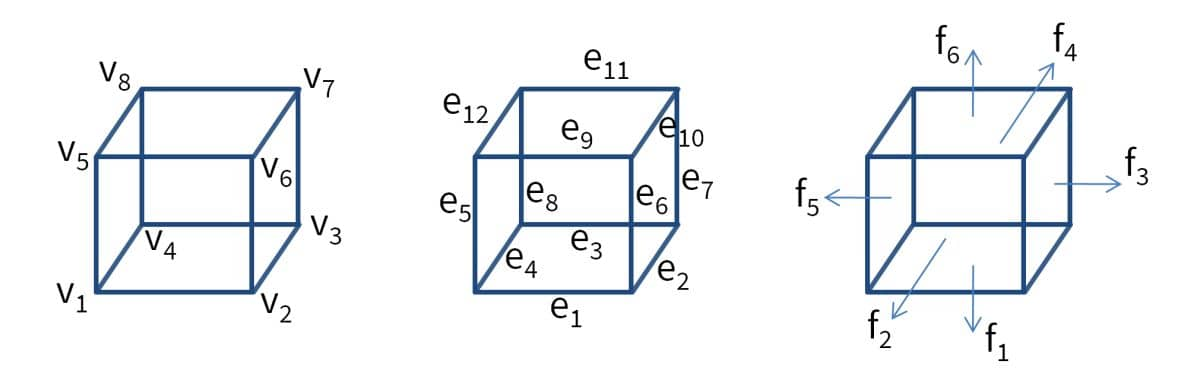## Grid Example 1

The following code can be used to manually define a one dimensional grid with 10 uniformly spaced cells on the line (0..1)

grid.p = 0:0.1:1;
grid.c = [1:10;2:11];
grid.a = [0:9;2:10 0];
grid.b = [1 10;1 2;1 2;-1 1];
grid.s = ones(1,10);


Alternatively one can use the grid utility function linegrid

grid = linegrid( 10, 0, 1 );


The plotgrid function can be used to plot and visualize a grid

plotgrid( grid )


## Grid Example 2

A 2 by 2 unit square rectangular grid can be created with the following commands

grid.p = [repmat([0,0.5,1],1,3);0 0 0 0.5 0.5 0.5 1 1 1];
grid.c = [1 2 5 4;2 3 6 5;4 5 8 7;5 6 9 8]';
grid.a = [0 2 3 0;0 0 4 1;1 4 0 0;2 0 0 3];
grid.b = [1 2 2 4 4 3 3 1;1 1 2 2 3 3 4 4;1 1 2 2 3 3 4 4; ...
0 0 1 1 0 0 -1 -1;-1 -1 0 0 1 1 0 0];
grid.s = ones(1,4);


The rectgrid function can also be used to create rectangular grids, in this case

grid = rectgrid( 2, 2, [0 1;0 1] );


As before the grid can be plotted, visualizing both grid point and cell numbers, with

plotgrid( grid, 'nodelabels', 'on', 'cellabels', 'on' )


Similarly, the boundaries can be visualized with the function plotbdr (subdomains can be visualized with plotsubd)

plotbdr( grid )


As FEATool also supports simplex triangular cells in two dimensions a grid consisting of quadrilaterals can easily be converted with the utility function quad2tri

grid = quad2tri( grid )


Reverse conversions can be made with tri2quad function which uses Catmull-Clark subdivision. In 3D the hex2tet and tet2hex functions perform similar conversions.

## Grid Example 3

A more complex example, a grid for a rectangle with a circular hole can be created by first creating geometry objects (a rectangle and a circle), applying a formula to construct the geometry shape, and then calling the automatic unstructured grid generation function gridgen

% Geometry definition.
xmin  = 0;
xmax  = 1;
ymin  = 0;
ymax  = 1;
tag1  = 'R1';
gobj1 =  gobj_rectangle( xmin, xmax, ymin, ymax, tag1 );

xc    = (xmax-xmin)/2;
yc    = (ymax-ymin)/2;
r     = 0.25;
tag2  = 'C1';
gobj2 =  gobj_circle( [xc yc], r, tag2 );

geom.objects = { gobj1 gobj2 };
formula = 'R1 - C1';
geom = geom_apply_formula( geom, formula );
fea.geom = geom;

% Grid generation.
hmax = 0.1;
fea.grid = gridgen( fea, 'hmax', hmax );


As before the grid can be plotted, visualizing both grid point and cell numbers, with

plotgrid( grid, 'nodelabels', 'on', 'cellabels', 'on' )


## Grid Import and Export

FEATool allows importing and exporting finite element grids between FEniCS, GiD, Gmsh, General Mesh Viewer (GMV), OpenFOAM, ParaView (VTK), and Triangle formats with the corresponding impexp_dolfin, impexp_gid, impexp_gmsh, impexp_gmv, impexp_foam, impexp_vtk, and impexp_triangle functions. These functions can also be accessed from the Grid and Postprocessing menus of the FEATool GUI.

Due to the simple grid format syntax it is possible to manually import grids from other software. The process essentially consists of first exporting the grid point coordinates and grid cell connectivity data from the external grid generation tool into separate text files. Then import them into MATLAB, after which they can be reshaped and used by FEATool. The following steps describe the process

1. The first step is to output the grid to an ASCII text format. If possible save the grid output to two files, one for the grid vertex coordinates, and another with the grid cell connectivities (this specifies which grid points/vertices make up each cell). If this is not possible one will have to manually open the grid output file in a text editor, and cut and save the grid coordinates and cell connectivities to two different files.
2. Load the two files in MATLAB (here it is assumed they are saved as p.txt and c.txt):
 load p.txt

3. Reshape the grid coordinates (p variable) so that it has the form $$n_{sdim}\times n_p$$ where $$n_{sdim}$$ is the number of space dimensions (1, 2, or 3) and $$n_p$$ is the total number of grid points (p essentially consists of rows of x, y, and z-coordinates). Precisely how to reshape depends on the output format from the external grid generator, one might not have to do anything (check the shape by entering the command size(p) or whos), it might be enough to transpose as p = p';, or one might have to reshape with something like p = reshape(p,n_sdim,n_p);.
4. Similarly, reshape the cell connectivity array c so that it has the shape $$n_v\times n_c$$ where $$n_v$$ is the number of vertices on each cell (for example 3 for triangles) and $$n_c$$ is the total number of cells. Each column should point to the corresponding grid points in p that make up the cell.
5. The grid vertices must run in counterclockwise order on each cell, so reorient them if necessary. This is usually accomplished by changing the row order, for example c = c([3 2 1],:); which the ordering for triangles.
6. Use the function gridadj to create an array that points to neighboring cells for each cell edge
 n_sdim = size(p,1);
a = gridadj( c, n_sdim );

7. Create a vector that assigns a subdomain number for each cell. If the geometry should be one single block and thus not split, a unit row vector is sufficient
 n_c = size(c,2);
s = ones( 1, n_c );

8. Use the function gridbdr to create boundary edge/face information. The gridbdr function splits external boundaries where the angles are acute
 b = gridbdr( p, c, a );

9. Alternatively, one can manually construct and set the boundary numbering, for example as

 [ix_edge_face,ix_cells] = find( a==0 );
b = [ix_cells'; ix_edge_face'; ones(1,length(ix_cells))];
b = [b; gridnormals(p,c,b)];


this creates a boundary array b where all external boundaries are joined. With additional boundary information one can now split the boundary edges and faces (columns) by assigning different boundary numbers (third row in b). A helpful function to use here is findbdr which allows one to find boundary numbers and column indices in to b by prescribing logical expressions such as x>0.5. For example

 [~,ix] = findbdr( struct('p',p,'c',c,'b',b), 'x>0.5', false );
b(3,ix) = 2;


sets boundary number 2 on all boundary edges/faces where x>0.5 is true.

10. Create a grid struct to hold all the grid information
grid.p = p;
grid.c = c;
grid.a = a;
grid.s = s;
grid.b = b;

11. Now the grid can be used by FEATool functions and subroutines on the command line.
12. Optionally, one can also add the grid to an existing finite element GUI model, by first exporting the FEA struct by using the File Menu option

 File > Export > FEA Problem Struct To Main Workspace


Set the grid field of the fea struct to the imported grid

 fea.grid = grid;


And re-import the fea struct into the GUI

 File > Import > Variables From Main Workspace...


Select the created fea variable to import and press Import. This loads it into the local FEATool memory workspace (note that this overwrites the existing fea data). Press the Grid mode button to update and show the new grid.

## Creating Structured Grids

The computational finite element library in FEATool supports FEM shape functions for structured grids (quadrilaterals in 2D and hexahedra in 3D). Although more difficult to generate automatically, structured grids are often computationally superior, allowing for higher accuracy with a smaller number of cells.

In FEATool, structured tensor-product grids of basic geometric shapes can easily be generated on the command line with the following MATLAB m-script functions

FunctionDescription
linegridCreate a 1D line grid
circgridCreate a 2D structured circular grid of quadrilateral cells
holegridCreate a 2D rectangular grid with a circular hole
rectgridCreate a 2D rectangular grid of quadrilateral cells
ringgridCreate a 2D grid of a ring shaped domain
blockgridCreate a 3D structured block grid
cylgridCreate a 3D structured cylindrical grid
spheregridCreate a 3D grid for a spherical domain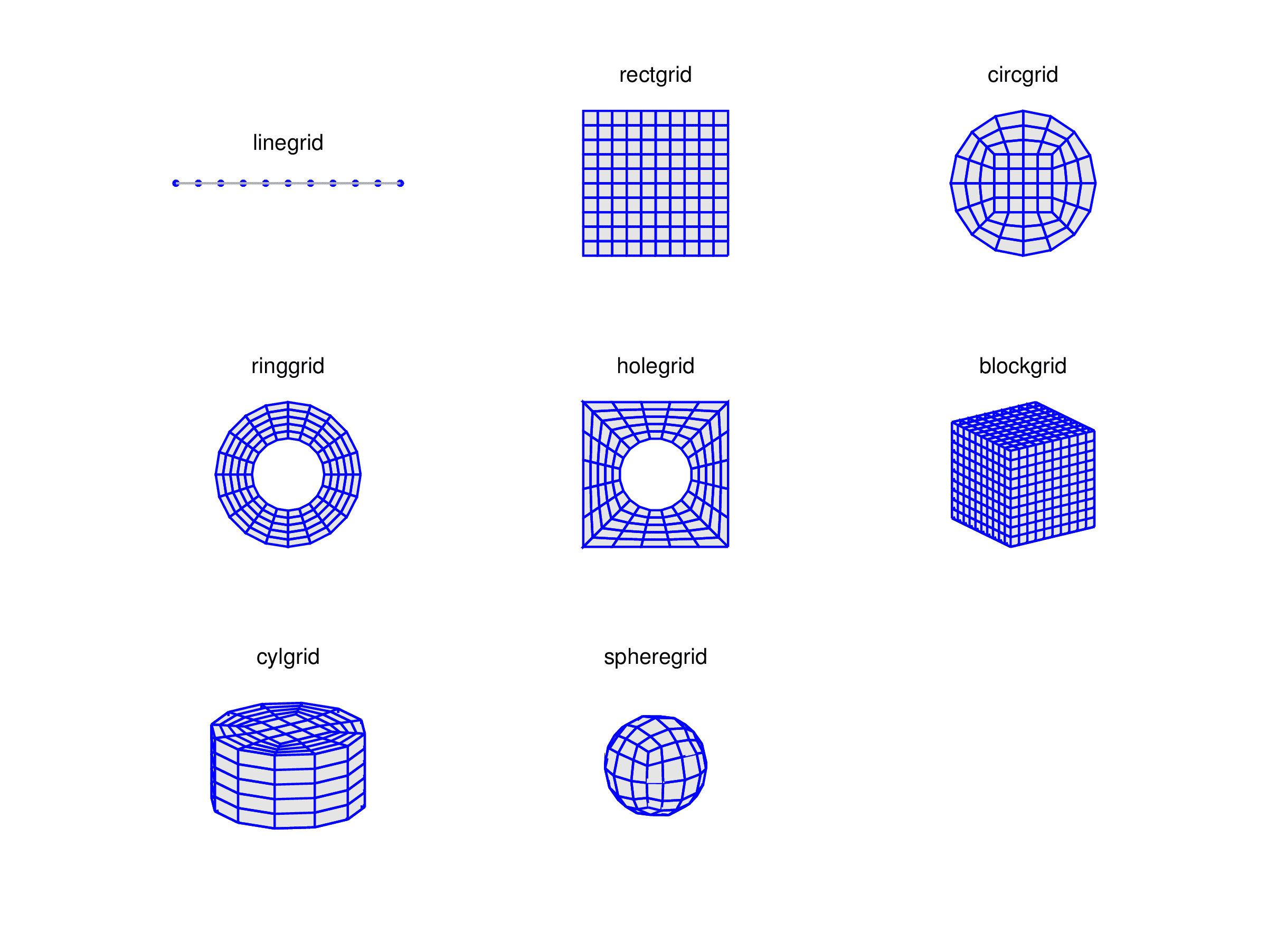Moreover, the grid utility functions selcells, delcells, gridextrude, gridmerge, gridrevolve, gridrotate, and gridscale can be used to manually modify, transform, and join grids to more complex structures.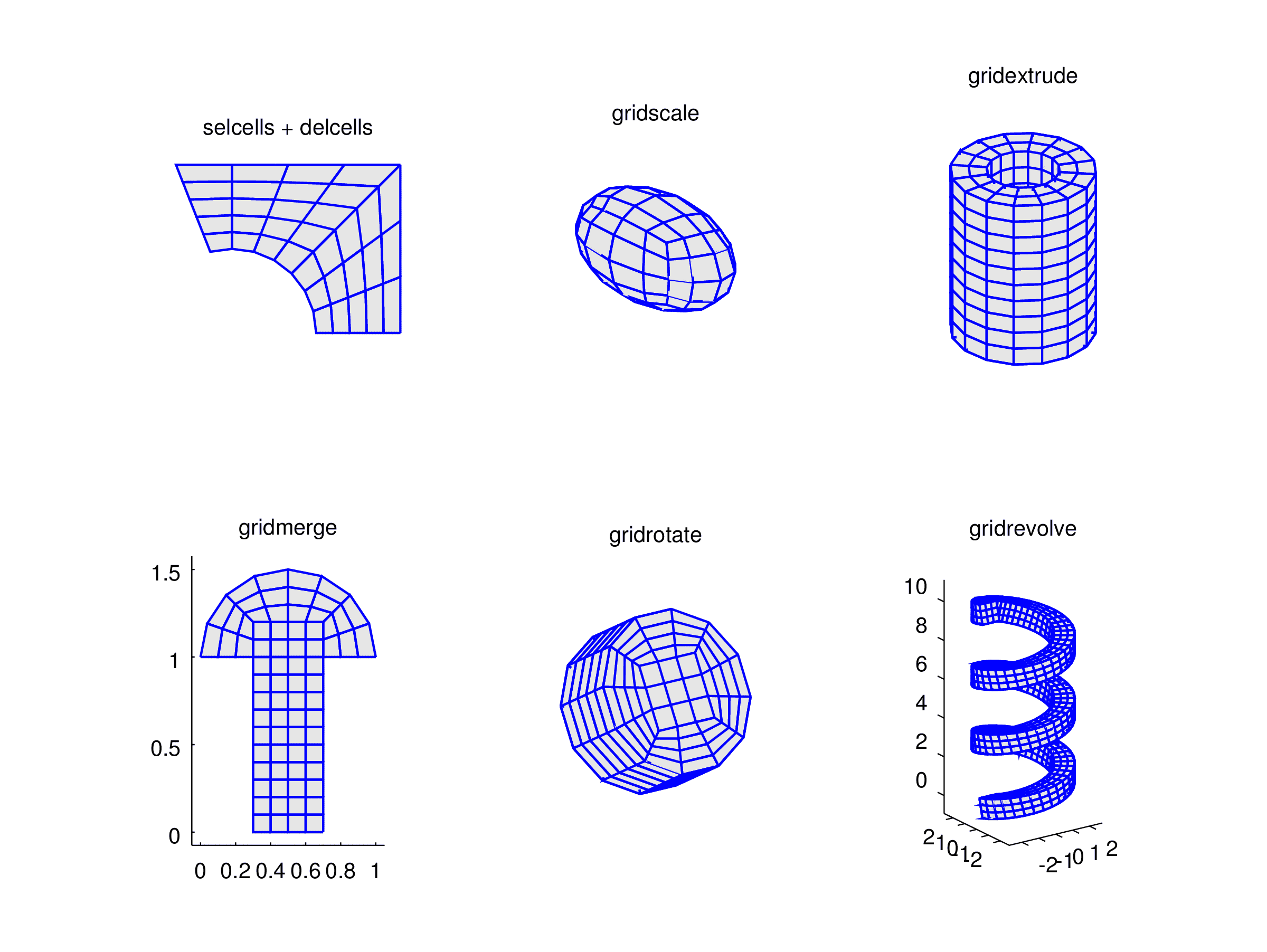The figure below shows three examples of more complex grids created by using these functions.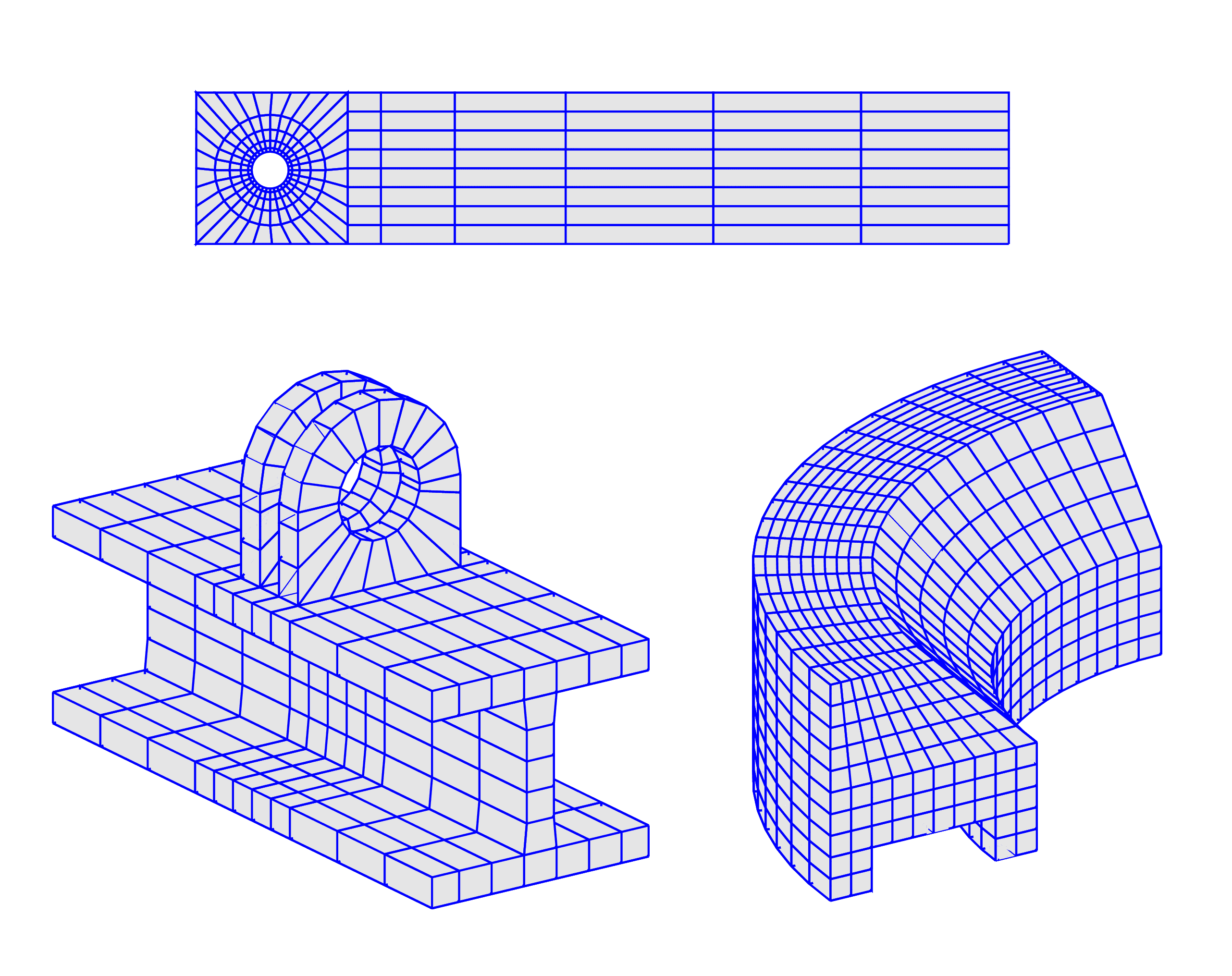The first flow over cylinder benchmark grid is for example created with the following commands:

 grid1 = ringgrid( [0.05 0.06 0.08 0.11 0.15], ...
32, [], [], [0.2;0.2] );
grid2 = holegrid( 8, 1, [0 0.41;0 0.41], 0.15, [0.2;0.2] );
grid2 = gridmerge( grid1, 5:8, grid2, 1:4 );
grid3 = rectgrid( [0.41 0.5 0.7 1 1.4 1.8 2.2], ...
8, [0.41 2.2;0 0.41] );
grid  = gridmerge( grid3, 4, grid2, 6 );


And the lower right revolved grid with these commands:

 grid = rectgrid();
grid = gridscale( grid, {'1-(y>0.5).*(y-0.5)' 1} );
grid = delcells( selcells( ...
'((x<=0.8).*(x>=0.2)).*(y<=0.2)', grid ), grid );
grid = gridrevolve( grid, 20, 0, 1/4, 2, pi/2, 0 );


The last example with two brackets attached to an I-beam section is more complex:

 grid01 = ringgrid( 1, 20, 0.03, 0.06, [0;0] );
indc01 = selcells( grid01, 'y<=sqrt(eps)' );
grid01 = delcells( grid01, indc01 );

grid02 = holegrid( 5, 1, .06*[-1 1;-1 1], .03, [0;0] );
indc02 = selcells( grid02, 'y>=-sqrt(eps)' );
grid02 = delcells( grid02, indc02 );
grid2d = gridmerge( grid01, [5 6], grid02, [7 8] );

grid1 = gridextrude( grid2d, 1, 0.02 );
grid1 = gridrotate( grid1, pi/2, 1 );
grid2 = grid1;
grid1.p(2,:) = grid1.p(2,:) + 0.03;
grid2.p(2,:) = grid2.p(2,:) - 0.01;

x_coord = [ -0.08 linspace(-0.06,0.06,6) 0.08 ];
y_coord = [ -0.2 -0.15 -0.1 -0.05 -0.03 -0.01 ...
0.01  0.03  0.05  0.1  0.15  0.2 ];
grid3 = blockgrid( x_coord, y_coord, 1, ...
[-0.08 0.08;-0.2 0.2;-0.08 -0.06] );
grid4 = blockgrid( 1, y_coord, 5, ...
[-0.01 0.01;-0.2 0.2;-0.18 -0.08] );
grid5 = grid3;
grid5.p(3,:) = grid5.p(3,:) - 0.12;

grid = gridmerge( grid1, 8, grid3, 6 );
grid = gridmerge( grid2, 8, grid, 19 );
grid = gridmerge( grid4, 6, grid, 24, 1 );
grid = gridmerge( grid5, 6, grid, 33, 2 );


After, the grids have been created on the command line they can also be imported into the FEATool GUI (by using the File > Import > Variables From Main Workspace option, after which the command fea.grid = grid; needs to be entered at the FEATool command prompt).

## Quadrilateral Grid Generation

Using quadrilateral grid cells are often advantageous to triangular cells in that they can provide somewhat more accuracy when aligned with geometry features and also tends to require less overall grid cells.

A mesh of quadrilateral grid cells can be created by using the gridgen function with quad as grid generation argument. This tries to align quadrilateral cell edges with immersed interfaces described by distance and level set functions. The algorithm then uses the zero level set contour from the distance functions to align grid cell edges with external geometry object boundaries. Furthermore, the cells are split in a way to treat edge cases such as when an interface segment crosses a cell diagonal. Optionally, the Gmsh mesh generator also allows for quadrilateral mesh generation. Finally, it is also possible to subdivide triangles and convert into quadrilaterals, however the resulting grids are often of poor quality and not suitable for physics simulations.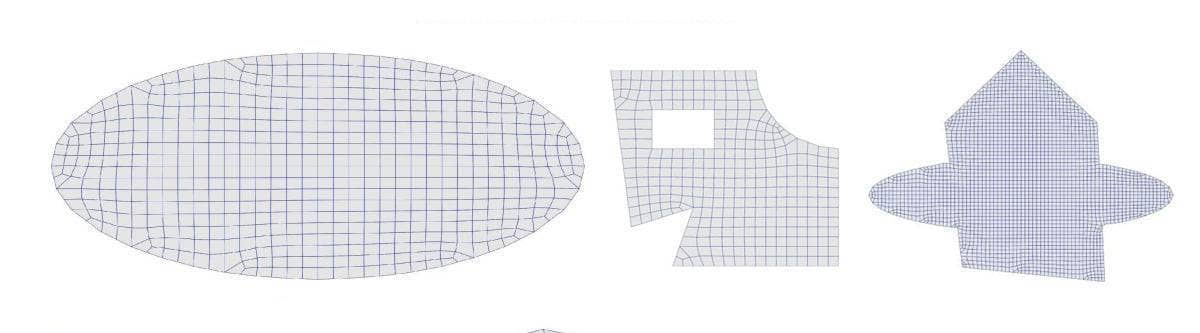## Grid Utility Functions

The following functions are available for working with and processing grids

FunctionDescription
gridgenUnstructured automatic grid simplex generation
gridrefineRefine a grid uniformly
gridstatShow grid statistics
gridsmoothApply smoothing to a grid
gridextrudeExtrude a grid from 2D to 3D
gridrevolveExtrude and revolve a 2D grid to 3D
gridrotateRotate a grid along a specified axis
gridscaleApply scaling to a grid
gridmergeMerge two grids along boundaries
gridnormalsDetermine normal vectors to external cell edges/faces
quad2triConvert a grid of quadrilateral cells to triangular cells
tri2quadConvert a grid of triangular cells to quadrilateral cells
hex2tetConvert a grid of hexahedral cells to tetrahedral cells
tet2hexConvert a grid of tetrahedral cells to hexahedral cells
impexp_dolfinImport and export FEniCS/Dolfin grid data
impexp_foamImport and export OpenFOAM grid data
impexp_gidImport and export GiD grid data
impexp_gmshImport Gmsh grid and postprocessing data
impexp_gmvImport and export GMV grid and postprocessing data
impexp_triangleImport and export 2D Triangle grid and polygon data
impexp_vtkImport and export ParaView VTK grid data
gridcheckCheck grid for errors